# Algebra 1 : How to subtract integers

## Example Questions

← Previous 1 3 4 5

### Example Question #1 : How To Subtract Integers

What is 4 – (–3)?

7

-1

-7

12

1

7

Explanation:

When subtracting integers, it is important to remember to add the inverse of the second number. In this case 4 – (–3), turns into 4 + (+3), which is 7.

4 – (–3)

4 + 3

7

### Example Question #1 : How To Subtract Integers

Evaluate the following: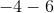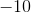Explanation:

When you subtract integers, it is the same thing as adding the inverse of the second integer. You can consider the following: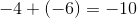You can also consider the problem as asking for six less than negative four. This will also get you to the answer of### Example Question #1 : How To Subtract Integers

Evaluate the following: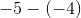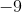Explanation:

When you subtract integers, it is the same thing as adding the inverse of the second integer. You can consider the following: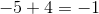### Example Question #3 : How To Subtract Integers

Simplify.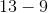Explanation:

This is a simple subtraction problem involving two positive numbers.is greater than; therefore, we will treat this operation as a normal subtraction problem.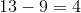The answer is.

### Example Question #4 : How To Subtract Integers

Simplify.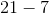Explanation:

This is a simple subtraction problem involving two positive numbers.is greater than; therefore, we will treat this operation as a normal subtraction problem.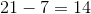The answer is.

### Example Question #2 : How To Subtract Integers

Simplify.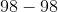Explanation:

This is a simple subtraction problem involving two positive numbers.

The numbers are the same with diferent signs; therefore, their difference is.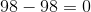### Example Question #6 : How To Subtract Integers

Simplify.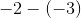Explanation:

This is a complex subtraction problem involving two negative numbers.Subtracting a negative number is the same as adding a positive number. Rewrite.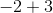Sinceis greater thanand is positive, the answer is positive. We will treat this operation as a subtraction problem.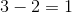The answer is.

### Example Question #7 : How To Subtract Integers

Simplify.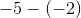Explanation:

This is a complex subtraction problem involving two negative numbers.Subtracting a negative number is the same as adding a positive number. Rewrite.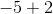Sinceis greater thanand is negative, the answer is negative. We will treat this operation as a subtraction problem.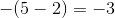The answer is.

### Example Question #8 : How To Subtract Integers

Simplify.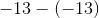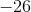Explanation:

This is a complex subtraction problem involving two negative numbers.Subtracting a negative number is the same as adding a positive number. Rewrite.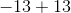The numbers are the same with diferent signs; therefore, their difference is.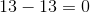### Example Question #9 : How To Subtract Integers

Simplify.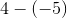Explanation:

This is a complex subtraction problem involving a positive and a negative number.Subtracting a negative number is the same as adding a positive number. Rewrite.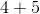We have an addition problem now.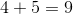The answer is.

← Previous 1 3 4 5